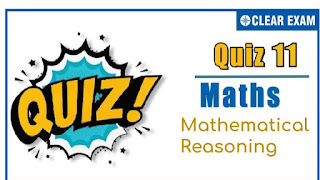## [LATEST]\$type=sticky\$show=home\$rm=0\$va=0\$count=4\$va=0

As per analysis for previous years, it has been observed that students preparing for JEE MAINS find Mathematics out of all the sections to be complex to handle and the majority of them are not able to comprehend the reason behind it. This problem arises especially because these aspirants appearing for the examination are more inclined to have a keen interest in Mathematics due to their ENGINEERING background.
Furthermore, sections such as Mathematics are dominantly based on theories, laws, numerical in comparison to a section of Engineering which is more of fact-based, Physics, and includes substantial explanations. By using the table given below, you easily and directly access to the topics and respective links of MCQs. Moreover, to make learning smooth and efficient, all the questions come with their supportive solutions to make utilization of time even more productive. Students will be covered for all their studies as the topics are available from basics to even the most advanced
.

Q1. If p: Ram is smart q: Ram is intelligent Then, the symbolic form Ram is smart and intelligent, is
•  (p∧q)
•  (p∨q)
•  (p∧∼q)
•  (p∨∼q)
Solution
(a) Given that p: Ram is smart q:Ram is intelligent The symbolic form of "Ram is smart and intelligent." is (p∧q)

Q2.The negation of p∧∼(q∧r) is
•  ∼p∨(q∧r)
•  ∼p∨(∼q∨∼r)
•  p∨(q∧r)
•  ∼p∨(q∧r)
Solution
(a)

Q3.  Switching function of the network is
•  (a∧b)∨c∨(a'b'c')
•  a∧b∨c∨(a'b'∧c)
•  a∨b∧c∧(a'b'c')
•  None of the above
Solution
Since, switches a and b and a', b' and c' are parallel which is denoted by a∧b and a'b'c' respectively Now, a∧b, c and (a'b'c') are connected in series, then switching function of complete network is a∧b∨c∨(a'b'c')

Q4. If p:A man is happy q:A man is rich Then, the statement, "If a man is not happy, then he is not rich" is written as
•  ~p → ~q
•  ~q → p
•  ~q → ~p
•  q → ~p
Solution
(a) ‘If a man is not happy, then he is not rich’ is written as ~p → ~q.

Q5.The statement (∼p∧q)∨∼q is
•  p∨q
•  p∧q
•  ∼(p∨q)
•  ∼(p∧q)
Solution
(d) ∼p∧q∨∼q≡∼q∨(∼p∧q) (By Commutative law) ≡∼q∨(q∧q∼p) (By Commutative law) ≡∼q∨q∼q∨∼p (By Distributive law) ≡∼(q∧p) ≡∼(p∧q)

Q6. The negation of q∨∼(p∧r) is
•  ∼q∨∼(p∧r)
•  ∼q∨(p∧r)
• ∼q∧(p∧r)
•  ∼q∧∼(p∧r)
Solution
(c) q∨∼p∧r=∼q∧(p∧r)

Q7.For any three propositions p,q and r, the proposition (p∧q)∧(q∧r)
•  p,q,r are all false
•  p,q,r are all true
•  p,q are true and r is false
•  p is true and q and r are false
Solution
(b) (p∧q)∧(q∧r) is true ⇒p∧q and q∧rare true (p and q are true) and (q and r are true) ⇒p,q and r are true

Q8.The statement p→(q→p) is equivalent to
•  p→(p↔q)
•  Tp→(p→q)
•  p→(p∨q)
•  p→(p∧q)
Solution
(b)

Q9.Which of the following sentences is a statements?
•  A Arushi is a pretty girl
•  What are you doing?
•  Oh! It is amazing
•  2 is the smallest prime number
Solution
(d)

Q10. Given that water freezes below zero degree Celsius. Consider the following statements : p: Water froze this morning, q: This morning temperature was below 0°C Which of the following is the correct?
•  p and q are logically equivalent
•  p is the inverse of q
•  p is the converse of q
• p is the contrapositive of q
Solution
(a) Clearly, p↔q## Want to know more

Please fill in the details below:

## Latest NEET Articles\$type=three\$c=3\$author=hide\$comment=hide\$rm=hide\$date=hide\$snippet=hide

Name

ltr
item
BEST NEET COACHING CENTER | BEST IIT JEE COACHING INSTITUTE | BEST NEET & IIT JEE COACHING: Reasoning QUIZ-11
Reasoning QUIZ-11
https://1.bp.blogspot.com/-2YseeUrNtbw/X9zxAQIVQZI/AAAAAAAAAEI/GwUM5HASUvMxiH1hr0W4yhanCFBIuLmWACLcBGAsYHQ/s320/QUIZ-11.jpg
https://1.bp.blogspot.com/-2YseeUrNtbw/X9zxAQIVQZI/AAAAAAAAAEI/GwUM5HASUvMxiH1hr0W4yhanCFBIuLmWACLcBGAsYHQ/s72-c/QUIZ-11.jpg
BEST NEET COACHING CENTER | BEST IIT JEE COACHING INSTITUTE | BEST NEET & IIT JEE COACHING
https://www.cleariitmedical.com/2020/12/reasoning-quiz-11.html
https://www.cleariitmedical.com/
https://www.cleariitmedical.com/
https://www.cleariitmedical.com/2020/12/reasoning-quiz-11.html
true
7783647550433378923
UTF-8